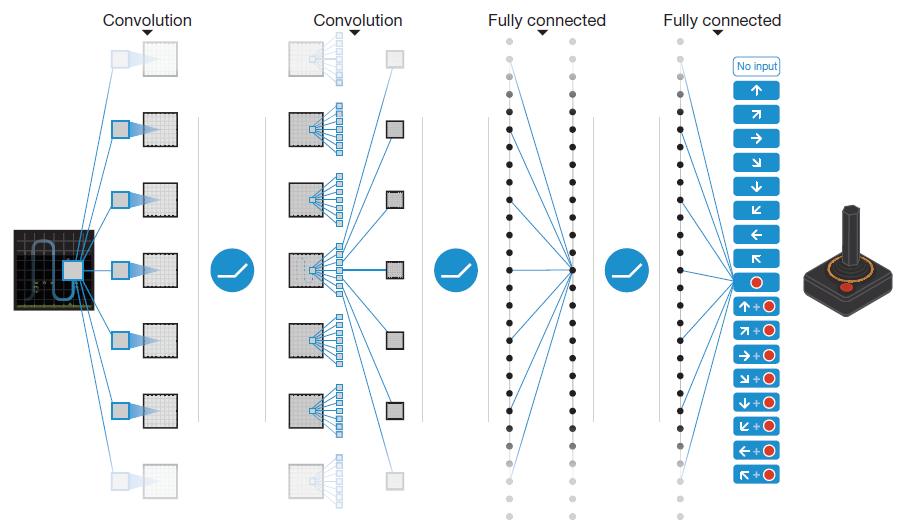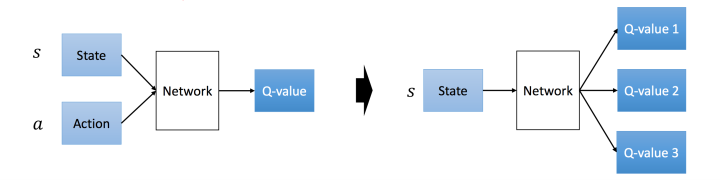# （强化学习）- DQN

DQN(Deep Q-)由 Google Deepmind 提出用于玩 Atair 系列的游戏以及 ALE 结构的游戏. DQN 的输入是一些像素点, 在过去这是很难被处理的. 同时一些强化学习的方法存在了一些挑战:

1. 深度学习的任务包含了大量的需要被标记的数据, 也就是说 RL 算法需要从那些标量的 Reward signal, 但是他们通常是稀疏的(很多 reward 都是 0), 有噪声的延迟的(与监督学习不同, action 和其造成的最终的回报的间隔时间很长(例如下棋)).
2. 深度学习算法是需要假设为独立的数据样本, 而在强化学习中状态可之间是有关系的(例如$S_{t+1}$是在$S_t$的状态下采取行动$a$转移到的).
3. DL 的目标分布固定; RL 的分布一直变化, 例如玩一个游戏,在一个关卡和下一个关卡的状态分布不同, 之前训练好的关卡又要重新训练.
4. 非线性网络表示值函数时, 出现不稳定等问题和发散(diverge)的问题

DQN 中解决这些问题的方法

• 通过 Q-learning 使用 reward 来构造标签(问题1)
• 为了解决相关的数据和不稳定分布(non-stationary distributions), 即问题2和3, 模型还引入了经验回放机制(experience replay mechanism).
• 使用 CNN(MainNet) 产生当前 Q值, 使用另一个 CNN(Target)产生 Target Q值.(问题4)

## 环境定义

agent 的交互环境为$\mathcal{E}$, 在时间$t$选择的行为 $a_t\in\mathcal{A}=\left\{1,\cdots,K\right\}$. 当前 agent 所处的状态是一个屏幕图像(即 observation)$x_t\in\mathbb{R}^d$, 当然, 一张图片无法使得 agent 了解到自己所处的状态(例如运动的方向以及速度), 所以我们定义一个 actions 和 observations 的序列

$$s_t=x_1,a_1,\cdots, a_{t-1},x_t$$

## Q-learning 的近似

$$Q^*(s,a)=\max_\pi\mathbb{E}[r_t+\gamma{r_{t+1}}+\gamma^2{r_{t+2}}+\cdots|s_t=s,a_t=a,\pi]$$

.$$\begin{equation} L_i(\theta_i)=\mathbb{E_{(s,a,r,s')}}\left[{\left({r+\gamma\max_{a'}Q(s',a';\theta_i^-)-Q(s,a;\theta_i)}\right)^2}\right] \label{loss_function_approx} \end{equation}$$

## 网络结构

.## 算法 & 模型部分

### 图像预处理

Atair 2600的每一帧是 210x160 的 128位彩色图, 所以函数$\phi$将一帧图像$x_t$进行裁剪, 输出84x84的图像, 同时将最近的四帧作为按照栈的顺序压入和弹出, 构成张量$s_t$.

### 算法

action 的空间已经定义为$\mathcal{A}$. 通常情况下, 环境$\mathcal{E}$是随机的(stochastic), 模拟器的内部状态是不可见的(有模拟系统控制实际的玩家), agent 智能观察图像. $r_t$ 将会反映在游戏得分的变化上, 有些游戏的分数取决于一系列的 actions 和 observations 序列之后. 所以这时候 action 的反馈有可能已经之后了很久了(time-steps have elapsed).

POMDP(部分观测的马尔可夫决策过程): 由于一帧画面并不能反应 agent 的状态, 所以我们使用了$s_t$ 来反应反应游戏的进行的状态. 这些序列(sequence, 指$s_t$)将会用于学习策略同时所有的序列将会在一个有限的 time step 终止, 所以就产生了巨大的有限马尔科夫决策过程(finite MDP).

$$Q^*(s,a)=\mathbb{E}_{s'}\left[{r+\gamma\max_{a'}{Q^*(s',a')}|s,a}\right]$$

#### Q-network

\begin{aligned} L_i(\theta_i)&=\mathbb{E_{s,a,r}}{\left[({\mathsf{E}\left[{y|s,a}\right]-Q(s,a;\theta_i)})^2\right]}\\ &=\mathbb{E_{s,a,r,s'}}{\left[{(y-Q(s,a;\theta_i))^2}\right]+\mathsf{E}_{s,a,r}[\mathsf{V_{s'}}[y]]} \end{aligned}